International Journal of Geomagnetism and Aeronomy
Vol 1, No. 2, November 1998

# A model of the electric field generated by viscous interaction in the magnetotail plasma sheet

N. V. Erkaev

Computing Center, Krasnoyarsk, Russia

### Abstract

A two-dimensional, time independent model of the quasi-viscous interaction of the magnetotail plasma sheet with the streaming solar wind is discussed in the low viscosity regime and with the assumption of a generalized Ohm's law for the electric current. The role of viscous forces is most significant in the boundary layers between the plasma sheet and solar wind, and these layers broaden in the downstream direction. A self-similar solution describing the distribution of velocity and potential in the boundary layers is obtained. The solution for the boundary layers determines the boundary conditions for finding the large-scale distribution of the electric potential in the plasma sheet.

### Introduction

Following Axford and Hines , models of quasi-viscous interaction of the magnetotail plasma sheet with the solar wind were also discussed by Krymskiy  and Pivovarov , who based their models on one-fluid MHD equations of the balance of viscous forces and Ampere forces.

For typical experimental values of the total current and magnetic field experiment, these models had to assume a rather large viscosity in the plasma sheet, which was difficult to justify physically.

This work presents a two-dimensional model of the quasi-viscous interaction in which the conductivity is high and the viscosity is low. The effect of the viscous forces is mainly concentrated in the boundary layers adjacent to the magnetopause. The generalized Ohm's law allowing for the electron pressure anisotropy is used for the electric current.

### Problem StatementConsider a semi-infinite plasma sheet (Figure 1) with dimensions 2d in the dawn-dusk direction and 2d in the north-south direction thick permeated by a magnetic field with a normal component B0. On the plasma sheet lateral walls adjacent to the solar wind are prescribed the plasma velocity u0 and electric potential F0 that depend on the interplanetary magnetic field (IMF), the origin of which is associated with reconnection or diffusion of the magnetic field at the magnetopause. Introduce the Cartesian coordinate system X, Y, Z, wherein the X axis is directed away from Earth, downstream, the Z axis is northward, and the Y axis is directed toward "dawn." We will consider the plasma stream only in the X, Y plane. In this case, the system of equations for ions has the form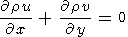The system of equations must be supplemented with the Ohm's law which, with allowance for drift currents, has the form+ (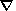xP/ (ne)) + b (yF + uB0 / c -yP/ (ne))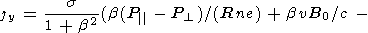- bxF + b (xP/ (ne)) -y F - uB0 / c +yP/ (ne))

Herex andy are the operators of differentiation with respect to x and y, s = ne2t/m is the plasma conductivity along the magnetic field, P| and Pare the field-aligned and transverse pressures, R is the field line curvature radius, n is the particle density, r = mpn is the mass density of ions, e is the electron charge, m is the electron mass, c is the speed of light, b = eB0t/mc is the magnetization parameter, t is the characteristic time of collision, u and v are the velocity components, F is the electric potential, and B0 is the magnetic field vector component normal to the neutral sheet, which we consider prescribed. The current density vector components are linked by the continuity equationSubstituting in the last equation the current density components defined from the Ohm's law yields the electric potential equation: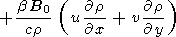Here W is the velocity vortex. Hereinafter, assuming for simplicity that the plasma density, pressure, and field line curvature are constant, we will be interested in the electric potential distribution and convection velocity field. In this case, the last equation becomes much simpler and takes the form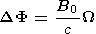(1)

In this context, taking into account the character of the assumptions made, we will be interested in the description of only a distant enough part of the magnetotail ( X > 20RE, where RE is the Earth's radius).

By cross differentiating the motion equations, one can eliminate the pressure and obtain the vorticity equation: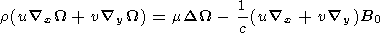Assuming that the magnetic field normal component is independent of x, y yields the following equation for the vorticity(2)

We introduce a current function y, in terms of which the velocity components are given by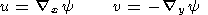(3)

Differentiating the last two equations yields the relationship of the current function and vorticity: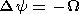(4)

Equations (1)-(4) form a closed system for the functions W, u, v, F , and y.

### Boundary Conditions

Consider the boundary conditions at the flanks of the plasma sheet, where it borders on the solar wind. We will assume that the velocity components are given here: the tangential component equals the solar wind speed, u = u0, and the normal component is zero, v = 0. Therefore the current function should meet the conditions at the boundary: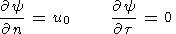The electric potential is also assumed to be given:

F|y=d = F0 F|y= - d = - F0

The equation is linear in relation to the electric potential; therefore solution for an arbitrary F can be presented in the form of the sum F = F+ F0y/d , where Fis a solution obtained with zero boundary condition for the potential, corresponding to a purely quasi-viscous interaction.

### Reduction to Dimensionless Form

Introduce dimensionless variables using the following normalizations:(5)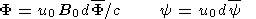(6)

Hereinafter we will omit the bar over the function designations for simplicity. In so doing, we will consider the variables made dimensionless by the above formulae. Changing to dimensionless variables yields the system of equations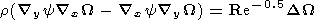(7)(8)

Here Re is the Reynolds number that depends on the viscosity coefficient: Re = u0d / m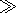1.

### Boundary Layer Method

Taking into account that Re1, we will solve the problem by the boundary layer method, which is widely used in calculations of viscous streams at large Reynolds numbers [Loitsyanskiy, 1978]. Let us express the unknown function in the form of a sum of rapidly varying and slowly varying functions:(9)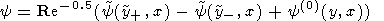(10)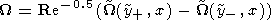(11)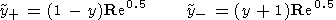(12)

The first two terms describe the boundary layers at the lateral boundaries of the plasma sheet at y=1 and y=-1.

Using an expansion of the solution of the type (9)-(12) and substituting it into the equation system yield, in the asymptotic limit Retwo systems. The first system allows determination of the boundary functions designated by the tilde. Since both boundary layers are absolutely symmetric, it will be enough to calculate one of these. To ensure determinacy, consider the equations of the boundary layer near the dusk boundary of the plasma sheet: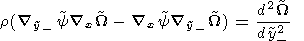(13)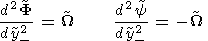(14)

The second system allows us to find a large-scale solution overlapping with the boundary one: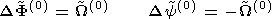(15)

Since the number of the sought functions has increased, additional boundary conditions are needed. First, boundary functions should meet the condition of approaching zero as the boundary layer variable tends to infinity: y+, y-: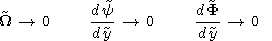(16)

At the boundaries at y=1 and y=-1, we have the conditions for the electric potential and velocity components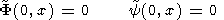(17)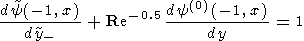(18)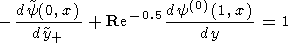(19)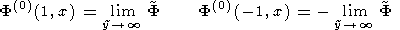(20)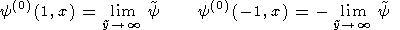(21)

In a zero approximation in the parameter Re-0.5, we have### Self-Similar Solution of Boundary Layer Equations

Introduce the self-similar variables

h = y / x x = y / x

f = F / x w = W / x

Then the boundary layer equations are transformed into ordinary differential equations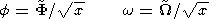Sought is a solution that meets the boundary conditions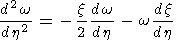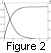Figure 2 shows the boundary layer functions obtained as a result of numerical integration of a self-similar system and satisfying the boundary conditions (16) and (19).

### Determination of Potential Outside Boundary Layers

Outside the boundary layers we have the Laplace equation

DF(0) = 0

Boundary conditions at the edges of the region under calculation are obtained from the conditions of asymptotic matching with the boundary layer solution:

F(0)(1, x) = ax F(0) (- 1, x) = - ax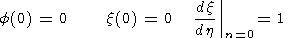Constant a is defined by numerical integration of equation system of the boundary layer.

With a view to obtaining an analytical solution, we will represent the potential in the form of the series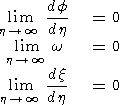which satisfies the Laplace equation on the condition that the expansion coefficients are linked by the recurrence relationship:The series absolutely converges at |y / x| < 1. Note that no boundary conditions at the section x=1 were discussed for the potential. Uncertainty of this condition in this problem is associated with the exclusion from discussion of the head section of the magnetosphere. In the general case, the correction to the above solution should satisfy the Laplace equation with zero conditions at y=-1, y=1 and the prescribed condition at x=1. The solution of such a problem decays quite fast with increasing x. Therefore, the effect of the additional condition should significantly weaken when moving away from Earth toward the magnetotail.

### Discussion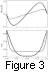Figure 3 shows the diagrams of variation of the electric potential and velocity in the dusk-dawn direction at a distance of x=2.5d. With d=20 RE, this distance is 50 RE The dashed lines correspond to Re = 1000, and the solid lines correspond to Re =100.The potential decreases in the dusk boundary layer from zero at the magnetopause to a minimum negative value and then increases nearly linearly to a positive maximum, and in the dawn boundary layer it again decreases to zero at the magnetopause. The maximum and minimum of the potential depend on the Reynolds parameter. According to Figure 3, the overall difference of potentials across the plasma sheet amounts to 0.16u0B0d/c at Re =1000 and 0.34u0B0d/c at Re =100. Assuming B0=1 nT, u0=400 km s -1, d=20 RE, and c=3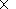1010 cm s -1, we will have numerical estimates of the difference of potentials: F max - F min8 kV at Re =1000 and F max - F min17 kV at Re =100. The points of turning to zero in the velocity coincide with the points of maximum and minimum electric potential. The distance between the magnetopause and the point of zero velocity is 0.2d at Re =1000 and 0.35d at Re =100. In the middle of the layer, plasma moves earthward at a speed of 0.1u0 at Re =1000 and 0.33u0 at Re =100. Figure 4a, b show the electric potential isolines in the plasma sheet plane. The coordinates are normalized to the half width of the plasma layer d.

Now we will try to estimate the Reynolds parameter. According to its definition, Re = ru0d / m. The viscosity coefficient m can be estimated by the formula rvtl, where vt is the thermal velocity of ions, and l is the free path length. If one assumes that the effective free path length is comparable in magnitude with the Larmor radius, then the following estimate can be obtained: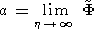where k is the Boltzmann constant, mp is the proton mass, vp is the thermal velocity of the proton, T is the temperature of proton in the plasma sheet, c is the speed of light, and e is the electron charge. Note that in the last formula the complete magnetic field modulus B should be substituted, which comprises a more significant Bx component and determines the Larmor radius.

Using the last equality and assuming [Akasofu and Chapman, 1972]

Tp5107 K       B = 10 nT

u0 = 400 km s-1       d = 20 RE

yields an estimate of the Reynolds parameter:### Conclusions

A two-dimensional model of the quasi-viscous interaction of the magnetotail plasma sheet with the streaming solar wind is discussed in the low viscosity regime. The role of viscous forces is most significant in the boundary layers that widen while receding downstream and are situated along the boundaries of the plasma sheet and solar wind. The motion of plasma in boundary layers permeated by the magnetotail magnetic field results in its polarization and occurrence of the dawn-dusk potential difference that depends on the effective turbulent viscosity. At a zero IMF and parameters of u0=400 km s -1 and B0=1 nT, the potential difference is 8-17 kV for Reynolds numbers 1000-100. In this context, the boundary layer thickness is 4 RE - 7 RE . At the internal boundaries of the boundary layers, the plasma velocity along the tail and the electric field across the tail are zero. Thus the boundary layer boundaries are the lines of changes in direction of plasma convection from tailward to earthward.

The Reynolds number of the order of 100 corresponds to the suggestion that the effective free path length of ions equals, in order of magnitude, their Larmor radius. With an IMF other than zero, an additional electric field associated with reconnection arises [Denisenko et al., 1992; Erkaev, 1988], which is summed with the quasi-viscous interaction field. For example, southward IMF reinforces earthward convection in the plasma sheet, and northward IMF suppresses it.

The theory discussed provides a good description of the physical processes involved and their interaction. However for the preferred value of Re =100, the boundary layer thickness is 7 RE and the earthward convection velocity in the central plasma sheet is ~130 km s -1. These values are rather large, especially since the convection speed would be increased for the case of southward IMF.

#### Acknowledgments

This work was supported by the International Science Foundation (project RKN300) and by the Russian Foundation for Basic Research (project 9505-14192).

### References

Akasofu, S.-I., and S. Chapman, Solar-Terrestrial Physics, 512 pp., Clarendon, Oxford, England, 1972.

Axford, W. I., and S. O. Hines, A unifying theory of high-latitude geophysical phenomena and geomagnetic storms, Can. J. Phys., 39, 1443, 1961.

Denisenko, V. V., N. V. Erkaev, A. V. Kitaev, and I. T. Matveenkov, Mathematical Modeling of Magnetospheric Processes, 197 pp., Nauka, Novosibirsk, 1992.

Erkaev, N. V., The results of research of MHD flow past the magnetosphere, Geomagn. Aeron., 28 (4), 529, 1988.

Krymskiy, P. F., A model of energy transfer in the Earth's magnetosphere, Issled. Geomagn. Aeron. Fiz. Sol., 62, 3, 1982.

Loitsyanskiy, L. G., Mechanics of Liquid and Gas, 736 pp., Nauka, Moscow, 1978.

Pivovarov, V. G., The effects of viscous interaction of the solar wind with the tail plasma sheet, Geomagn. Aeron., 5, 876, 1981.

Load files for printing and local use. This document was generated by TeXWeb (Win32, v.2.0) on November 4, 1998.